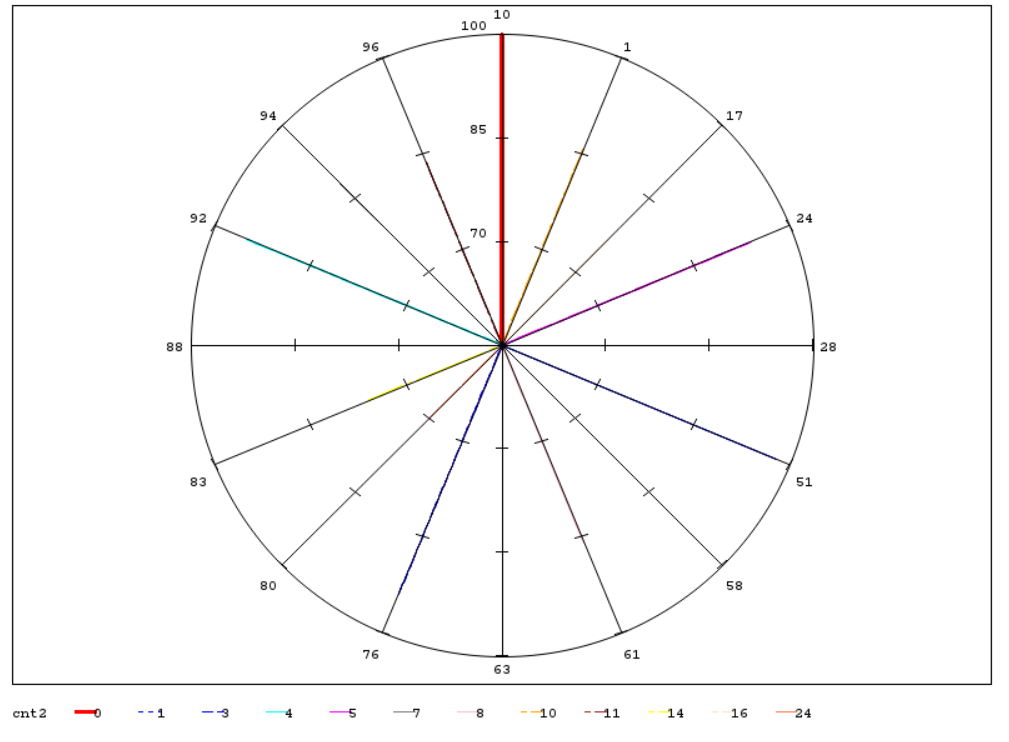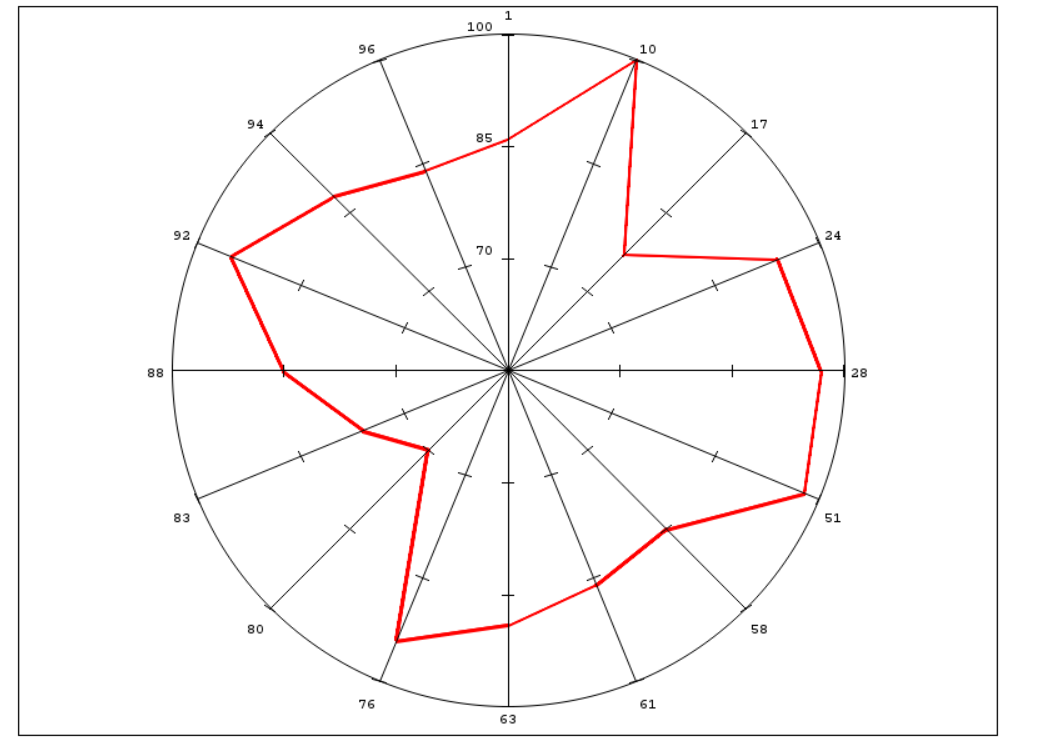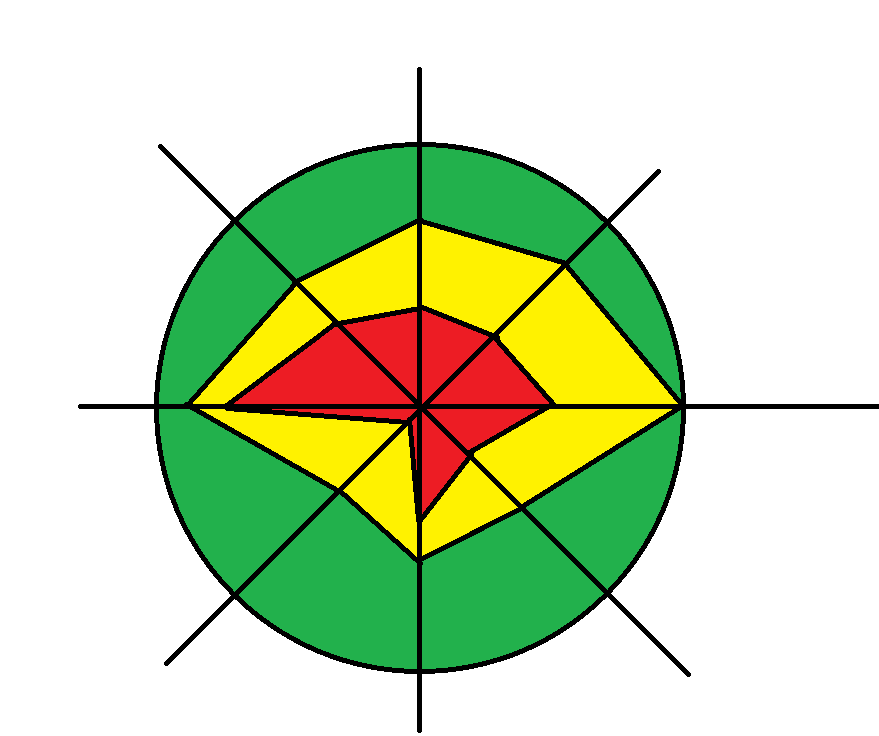Good day!

I have a problem with gradar again.

This is my data:

viewcnt1cnt2cnt3id
1871041
21000010
37716617
4955024
5973028
6991051
7867758
8878561
9897463
10953276
117024680
127714983
138611388
14964092
15887594
168511596

This is my code:

```data test;
set test;
format cnt1 cnt2 cnt3 3.0;
run;

ods _all_ close;

goptions device=png;
ods HTML STYLE=&_ODSSTYLE. nogtitle nogfootnote body=_WEBOUT(NOTOP NOBOT) path=&_TMPCAT. (url=&_REPLAY.) ;

chart id /  freq=cnt1
overlayvar=cnt2
cstars=(red, blue)
wstars=2
lstars=1
starcircles=(1.0);
run;
quit;

ods HTML close;
```

This is what happen when I use overlayvar in gradar:Separately variables drawn perfect:I try do something like this:I assumed there is a problem with zero value: I tried without zero but result was the same.

1 ACCEPTED SOLUTION

Accepted Solutions

## Re: Gradar: two variables problem.

Hi,

First you should rearrange (transpose) your data.

Look at the example in the user's guide:

SAS/GRAPH(R) 9.2: Reference, Second Edition

the structure of the data is here:

SAS/GRAPH(R) 9.2: Reference, Second Edition

In your case the new dataset should contain the following variables: id, cnt, <overlayvar> (with values 1, 2, 3)

2 REPLIES 2

## Re: Gradar: two variables problem.

Hi,

First you should rearrange (transpose) your data.

Look at the example in the user's guide:

SAS/GRAPH(R) 9.2: Reference, Second Edition

the structure of the data is here:

SAS/GRAPH(R) 9.2: Reference, Second Edition

In your case the new dataset should contain the following variables: id, cnt, <overlayvar> (with values 1, 2, 3)

## Re: Gradar: two variables problem.

Thank you so much!

Discussion stats
• 2 replies
• 781 views
• 0 likes
• 2 in conversation